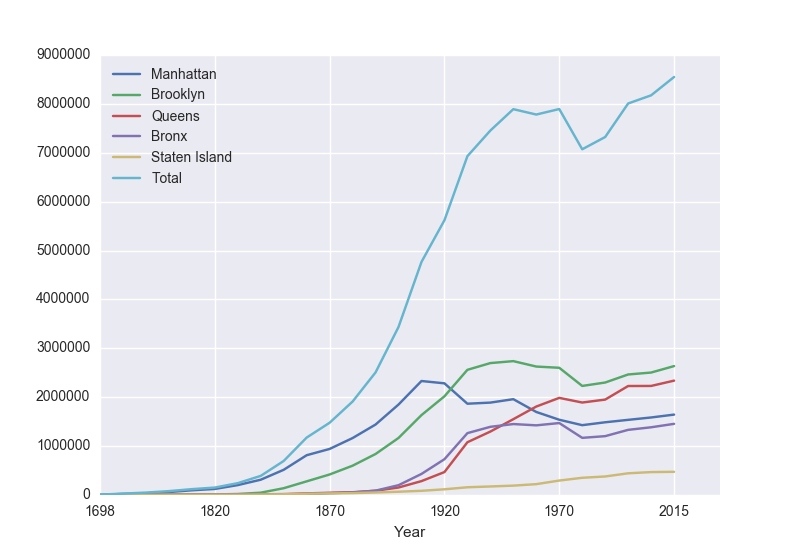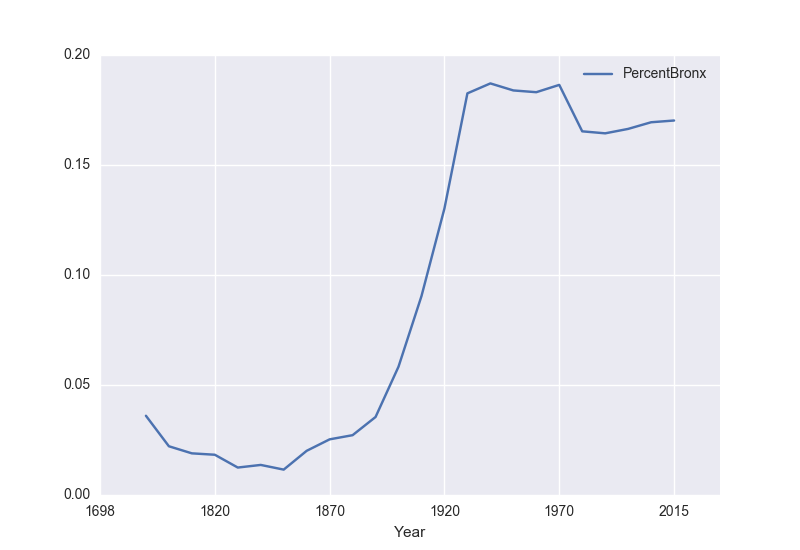Today's lab will focus on looping through data files and using decisions.
Software tools needed: web browser and Python IDLE programming environment.

To make reading files easier, we will use the pandas library that lets you read in CSV files very efficiently. Pandas, Python Data Analysis Library, is an elegant, open-source package for extracting, manipulating, and analyzing data, especially those stored in 2D arrays (like spreadsheets). It incorporates most of the Python constructs and libraries that we have seen thus far.

Pandas should be installed on all the lab machines. If you are using your own machine, you should check that it is there by typing at the Python shell (in spyder, idle, or your favorite Python interface):

import pandas as pd

If you get an error that the library is not found. Open up a terminal, and use conda to install it:

conda install pandas
In Pandas, the basic structure is a DataFrame which stored data in rectangular grids. Let's use this to visualize the change in New York City's population. First, start your file with an import statements for pandas:
import pandas as pd
The as pd allows us to use pandas commands without writing out pandas everytime-- instead we just write pd.

Next, save the NYC historical population data to the same directory as your program. Open the file in your favorite spreadsheet program (such as Excel) to see what it looks like. Note that it has 5 extra lines at the top before the column names occur. The pandas function for reading in CSV files is read_csv(). It has an option to skip rows which we will use here:

Before going on, let's print out the variable pop. pop is a dataframe, described in the reading above:

print(pop)
The last line of our first pandas program is:
pop.plot(x="Year")
which makes a graphical display of all of the data series in the variable pop with the series corresponding to the column "Year" as the x-axis. Your output should look something like:To recap: the 3-line program:

import pandas as pd
pop.plot(x="Year")

If you are using IDLE, you will need two more lines to make plots pop up automatically:

import matplotlib.pyplot as plt

import pandas as pd
pop.plot(x="Year")

plt.show()
did the following:
• Imported the pandas library that contains structures and functions for organizing and visualizing data. (The additional line explicitly imports the pyplot library which pandas uses to create figures.)
• It read in a CSV file, containing NYC population historical data.
• It displayed the data as a visual plot of years versus borough populations.
• The last line in the version for IDLE shows the figure you created (spyder automatically displays it; in IDLE, you need to request that the plot you created be shown).
There are useful built-in statistics functions for the dataframes in pandas. For example, if you would like to know the maximum value for the series "Bronx", you apply the max() function to that series:
print("The largest number living in the Bronx is", pop["Bronx"].max())
Similarly the average (mean) population for Queens can be computed:
print("The average number living in the Queens is", pop["Queens"].mean())

Challenges

• What happens if you leave off the x = "Years"? Why?
• What happens if you add in x = "Years", y = "Bronx"?
• What does the series functions: .min(), .std(), and .count() do?

Manipulating Columns

Each column in the original spreadsheet is a column, or series. We can look at the column for the Bronx with:

print(pop['Bronx'])
How would you look at the one for Brooklyn?

A nice thing about series is that you can do basic arithmetic with them. For example,

print(pop['Bronx']*2)
prints out double the values in the column.

You can also use multiple columns in a calculation:

print(pop['Bronx']/pop['Total'])
prints out the fraction of the total population that lives in the Bronx.

We can save that series by creating a new column for it:

pop['FractionBronx'] = pop['Bronx']/pop['Total']
and then can use it to create a new graph:
pop.plot(x = 'Years', y = 'FractionBronx')
shown in the following plot:Using NYC OpenData

Much of the data collected by city agencies is publicly available at NYC Open Data. Let's use pandas to plot some data from NYC OpenData.

We'll start with data that has the daily number of families and individuals residing in the Department of Homeless Services (DHS) shelter system:

Click on the "View Data" button. To keep the data set from being very large (and avoid some missing values in 2014), we are going filter the data to be all counts after January 1, 2015. To do this:

• Click on the "Filter" button.
• On the menu that pops us, click on "Add a New Filter Condition".
• Choose "Date of Census" but change the "is" to be "is after".
• Click in the box below and a calendar will pop up. Highlight January 1, 2015.
• Click the check box to the left of the data.
• It will take a few seconds (it's a large file) but the rows on the left will be filtered to be all counts after January 1, 2015.
• Click on the "Export" button.

Move your CSV file to the directory you save your programs. Open with Excel (or your favorite spreadsheet program) to make sure it downloaded correctly. Look at the names of the columns since those will correspond to series we can plot.

Now, we can write a (short) program to display daily counts:

import pandas as pd

homeless.plot(x = "Date of Census", y = "Total Individuals in Shelter")